Electricity & Megnetism

# Association of capacitors

Capacitor association is the way of connecting two or more capacitors to obtain different capacitances. Basically there are two types of association between capacitors: series or parallel, however it is possible to combine them, forming mixed associations. The study of capacitor associations represents an important part of electrodynamics and electrical circuits .

## Capacitors

Capacitors are small electronic devices used primarily to store electrical charges . The basic structure of a capacitor, regardless of its type, is composed of two metallic armatures , separated by some dielectric material , such as paper, oil, rubber, etc. The dielectric serves to make it difficult for charges to pass between the capacitor terminals. The greater the electrical resistance of this material, the greater the amount of charge accumulated by the capacitor.

Currently, capacitors are manufactured in varying specifications, however, many circuits combine them in series or in parallel. By doing this, it is possible to obtain capacitance values ​​according to the needs of each electrical circuit, in the case where capacitors with the desired characteristics are not manufactured, for example. If you want to know more about these devices, read: Capacitors .

capacitance

The main characteristic of capacitors is capacitance — a physical quantity that measures the amount of charge that a capacitor can store for a given potential difference . The unit of measurement for capacitance is the farad (F), where 1 farad is equivalent to 1 coulomb per volt (C/V) . The capacitance formula is this: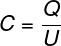C – capacitance (F)

Q – electrical charge (C)

U – electrical voltage (V)

If you want to delve into the topic of this topic, read: Capacitance of a capacitor .

## Types of capacitor association

Let’s get to know the characteristics of associations between capacitors and the formulas used to calculate the equivalent capacitance in each case:

• ### Combination of capacitors in series

In the association of capacitors in series, all capacitors are connected to a single wire, thus, the amount of electrical charges stored in each capacitor is equal. In the figure below, we have a series association of five capacitors, note:

In this type of association, it is possible to obtain the equivalent capacitance through the sum of the inverses of the individual capacitances, check: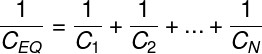EQ – equivalent capacitance (F)

For the case of two capacitors associated in series, of capacitances C 1 and C 2 , it is possible to use the product by sum rule , however, it is necessary to remember that this rule can only be used for every two capacitors associated in series, note :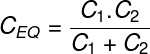In the following figure, we schematize an electrical circuit in which five capacitors are associated in series and connected to a battery and a switch.

• ### Connecting capacitors in parallel

In the association of capacitors in parallel, all capacitors are subjected to the same potential difference , in addition, the individual capacitances add up, so the equivalent capacitance is equal to the sum of the capacitances. This figure shows a circuit of three capacitors in parallel, check it out:

The equivalent capacitance of the association of capacitors in series is made by adding the capacitances, check the formula for N capacitors: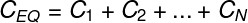In the next figure, we schematize an electrical circuit composed of three capacitors connected in parallel, a battery and a switch:

• ### Mixed association of capacitors

A mixed combination of capacitors is one in which, in the same electrical circuit, there are capacitors connected both in series and in parallel. In this case, it is necessary that we first solve those capacitors that are connected directly in series or in parallel, in order to reduce the number of associations.

## Capacitor association exercises

Question 1) Two capacitors, each of 1 mF, are connected in series. The equivalent capacitance of the association corresponds to:

a) 0.75 mF

b) 1.5 mF

c) 0.5 mF

d) 2.0 mF

e) 2.5 mF

Template: Letter C

Resolution:

To find the value of the equivalent capacitance of the capacitors associated in series, let’s use the product by sum rule, check: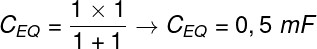Based on the calculation, we found that the equivalent capacitance of this association is equal to 0.5 mF, so the correct alternative is the letter C .

Question 2) Three capacitors, with capacitances equal to 0.5 nF, 1.5 nF and 3.0 nF, are connected in parallel. The equivalent capacitance of such an association is equal to:

a) 0.5 nF

b) 5.0 nF

c) 1.2 nF

d) 0.3 nF

e) 1.8 nF

Template: letter B

Resolution:

In the case of parallel association, we just add the equivalent capacitances, so the correct answer is the letter B .

Question 3) About the association of capacitors in parallel, mark the CORRECT alternative:

a) All capacitors have the same electrical charge.

b) The equivalent capacitance is always less than any of the individual capacitances.

c) Capacitors are subjected to different electrical voltages.

d) All capacitors are subjected to the same electrical voltage.

e) None of the alternatives.

Template : Letter D

Resolution:

In the association of capacitors in parallel, all capacitors are subject to the same electrical voltage, so the correct answer is the letter D .